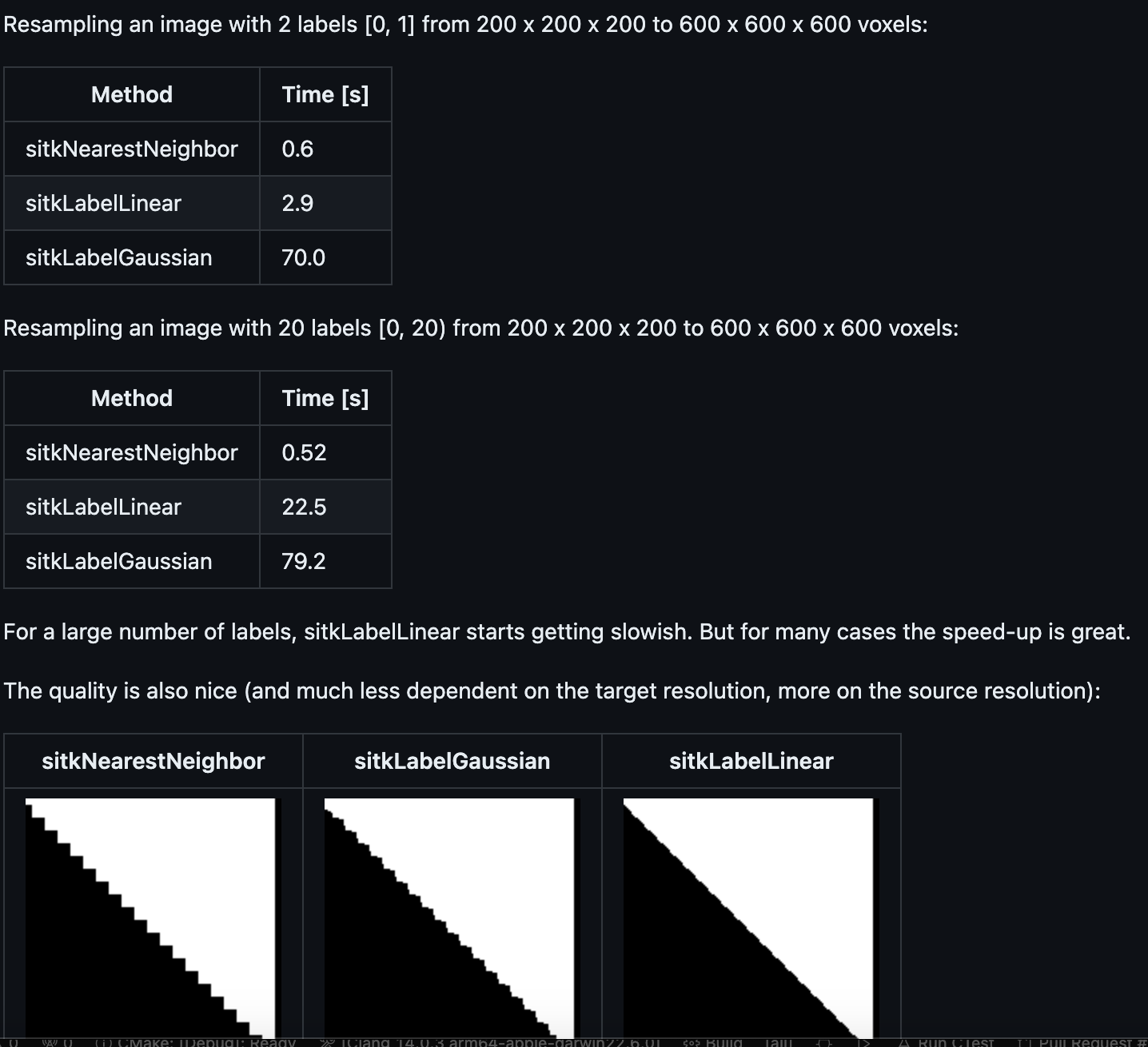# Faster interpolation using sitkLabelGaussian

Hi

I am working on a MONAI-based whole head segmentation tool, which predicts close to 30 tissue labels.

Currently all post-processing is implemented in Python using SimpleITK. Once we have done some clean-up operations at 1mm resolution, we need to insert thin layers of Dura and Cerebrospinal fluid. For this purpose we resample the segmentation to 0.3-0.5mm using the `ResampleImageFilter` (sitkLabelGaussian option).

Unfortunately, this gets very slow (it is using all CPUs). Is there any way to get smooth interpolation (i.e. not nearest neighbor) but better performance. I doubt computing the signed distance transform and smoothing for each label will scale nicely (approx. 30 labels).

To implement a similar behavior, we could

• convert labels to one-hot (e.g. 10 channels for labels in range [0, 9]).
• smooth the one-hot “vector” image
• resample the smoothed on-hot image
• perform argmax to get resampled & smoothed labels

The problem is that this will explode memory usage (at least GPU)). For 600x600x600 images, with 30 labels, using 32-bit float we would need 24 GB (600 x 600 x 600 x 30 x 4 B) per one-hot image.

``````def test_LabelGaussianInterpolation():
"""Example code implementing the label gaussian with MONAI/pytorch"""
labels = sitk.Image(100, 110, 80, sitk.sitkUInt16)
labels[:] = 0
labels[3:40, 80:90, 25:60] = 1
labels[15:35, 30:95, 50:70] = 2
labels[30:35, 30:35, 30:35] = 3

num_classes = 4
file_path = Path.cwd() / "labels.nii.gz"
sitk.WriteImage(labels, file_path)

to_tensor = EnsureType(dtype=torch.half)
to_one_hot = AsDiscrete(to_onehot=num_classes, dtype=torch.half)

labels_tensor = to_tensor(labels_tuple)
log_tensor("input", labels_tensor)

onehot_tensor = to_one_hot(labels_tensor)
log_tensor("onehot", onehot_tensor)

smooth = GaussianSmooth(sigma=1.5)
smooth_onehot_tensor = smooth(onehot_tensor)
log_tensor("smooth", smooth_onehot_tensor)

resample = Spacing(pixdim=0.5)
hires_onehot_tensor = resample(smooth_onehot_tensor)
log_tensor("hires", hires_onehot_tensor)

to_argmax = AsDiscrete(argmax=True)
hires_argmax_tensor = to_argmax(hires_onehot_tensor)
log_tensor("argmax", hires_argmax_tensor)

saver = SaveImage(
resample=False,
output_postfix="hr",
output_dir=Path.cwd(),
separate_folder=False,
)
saver(hires_argmax_tensor)
``````

To solve the memory issue, we could maybe use 16-bit float (torch.half). A much more drastic saving would be to iterate over the channels, and update the current maximum of the smooth indicator functions and corresponding label.

Note: Of course the CPU memory might be enough, but then the performance will not be better than the current sitkLabelGaussian.

The above may more efficiently be implemented in SimpleITK/ITK using the LabelMap representation. The LabelMap “Images” a represented as sparse RLE. Each label could be extracted the smoothing/resampling performed and then converted to a LabelMap and merged together.

Alternatively consider using morphological operations to smooth the results after nearest neighbor interpolation? Performing opening and closing may yield acceptable results.

There is a ITK remove module ITKLabelErodeDilate which efficiently operates on multi-labeled images. I demoed it once a while ago, but have not used it recently.

The author of the module @richard.beare who may have more information on if its suitable for this application.

1 Like

Thanks for the suggestion @blowekamp!

I have used the LabeErodeDilate module quite a lot. I guess you are proposing to:

• convert to LabelMap representation
• smooth each label
• merge with some priority (for overlapping voxels)
• fill holes with the LabelSetDilateImageFilter (from LabeErodeDilate), or with my ITKDissolve module

I will give it a try and see what is faster.

Have you looked at the more general GenericLabelInterpolator where you can substitute the linear analog? The related IJ article shows evidence improvement in terms of both accuracy and computational performance over the Gaussian version that I wrote. For what it’s worth, it’s what we’ve been using in ANTs for years.

1 Like

True. Thanks @ntustison! I have only used the GenericLabelInterpolator from C++ but I see could try the GenericLabelInterpolator via the python remote module.

Maybe I will create a PR to add it as sitkLabelLinear to SimpleITK.

2 Likes

The contribution will be most welcomed.

2 Likes

@ntustison thanks for the GenericLabelInterpolator suggestion. The speed-up is not bad, especially if the labelmap doesn’t have too many labels.4 Likes

Interesting. Thanks for posting this.

1 Like